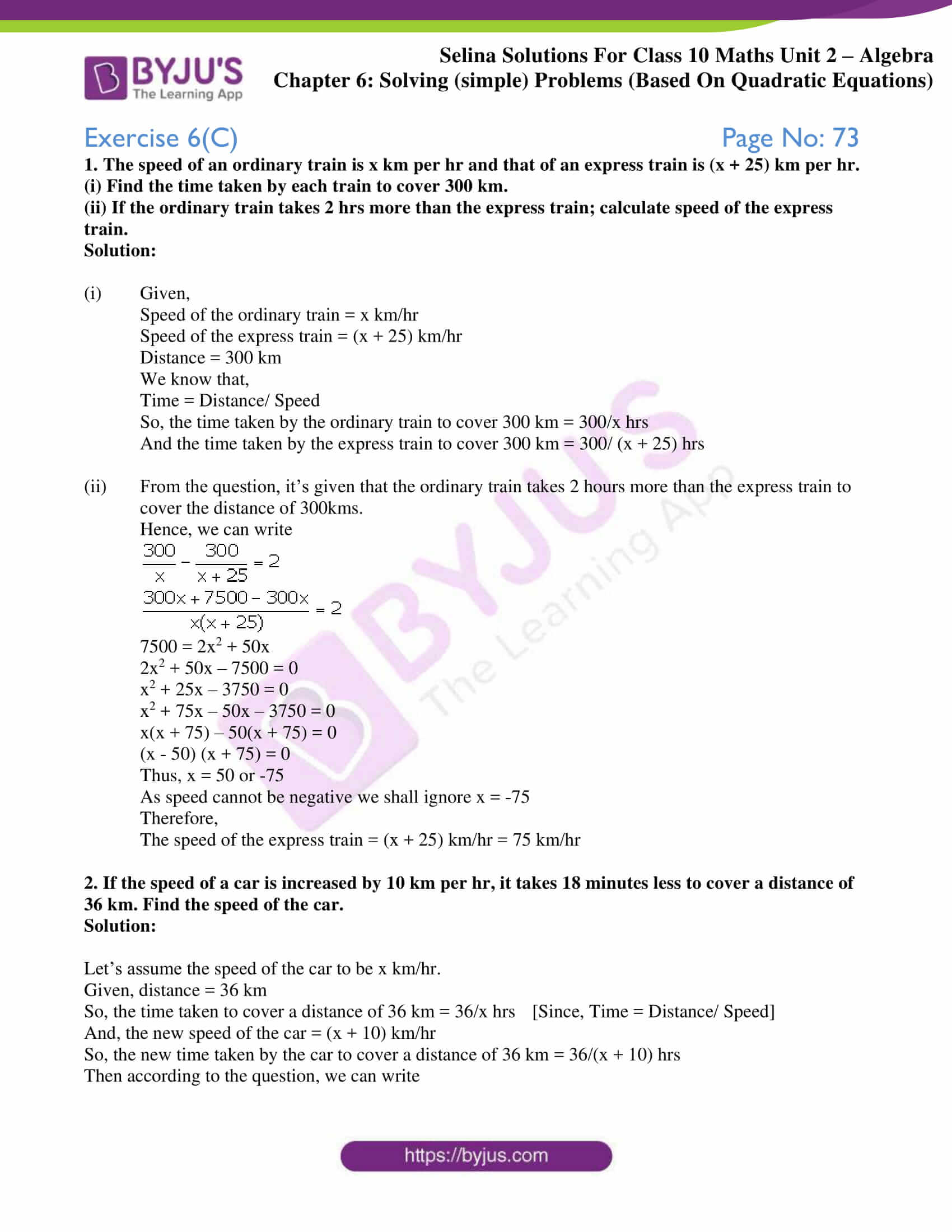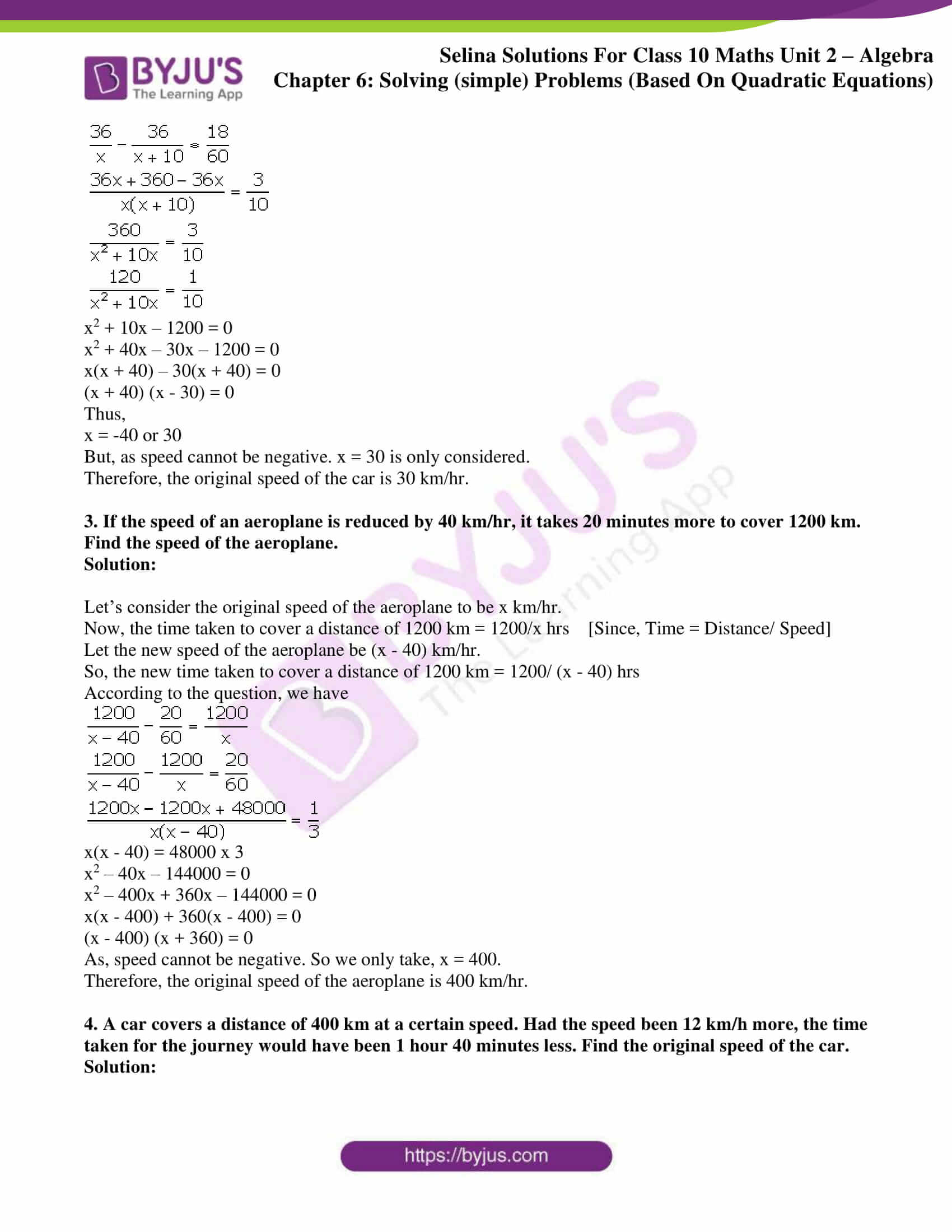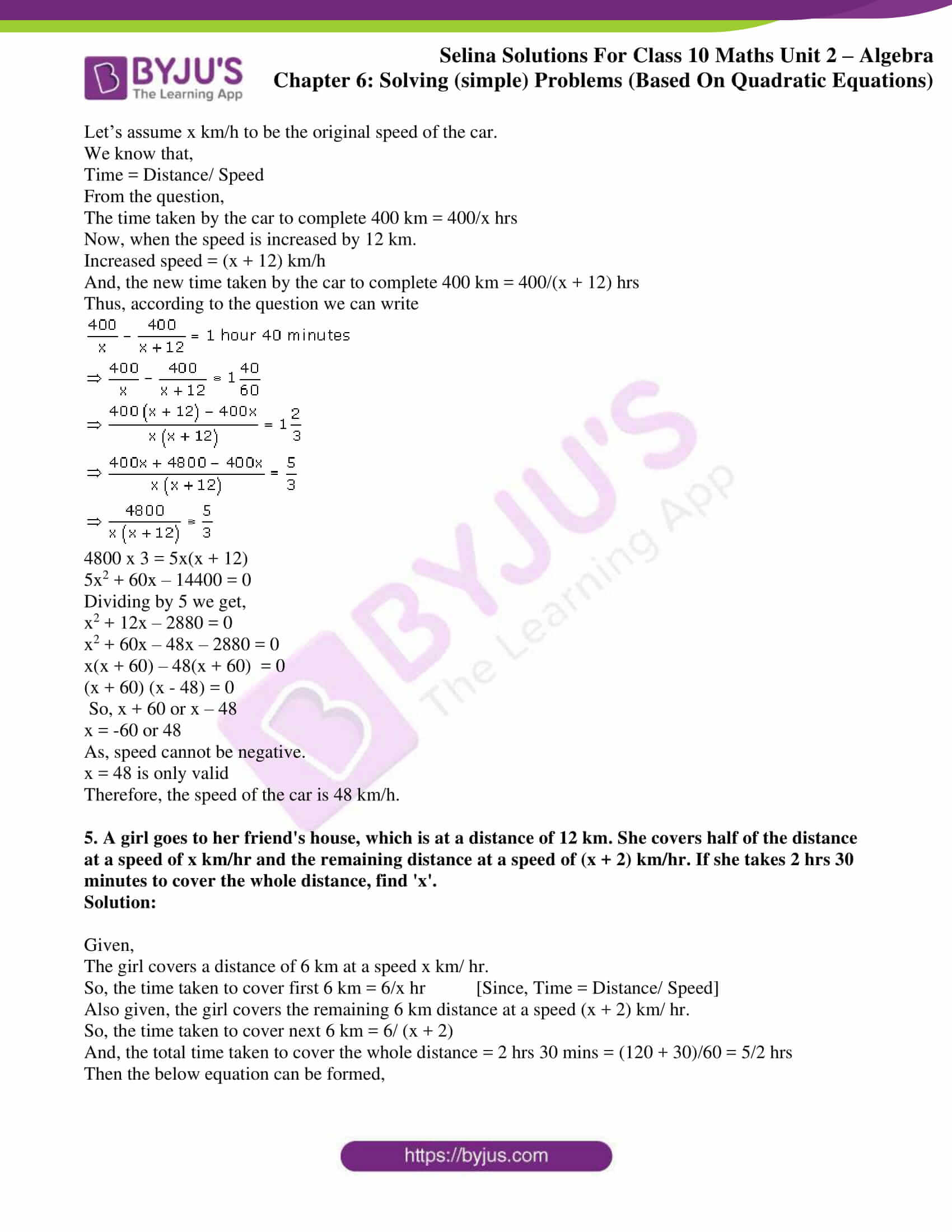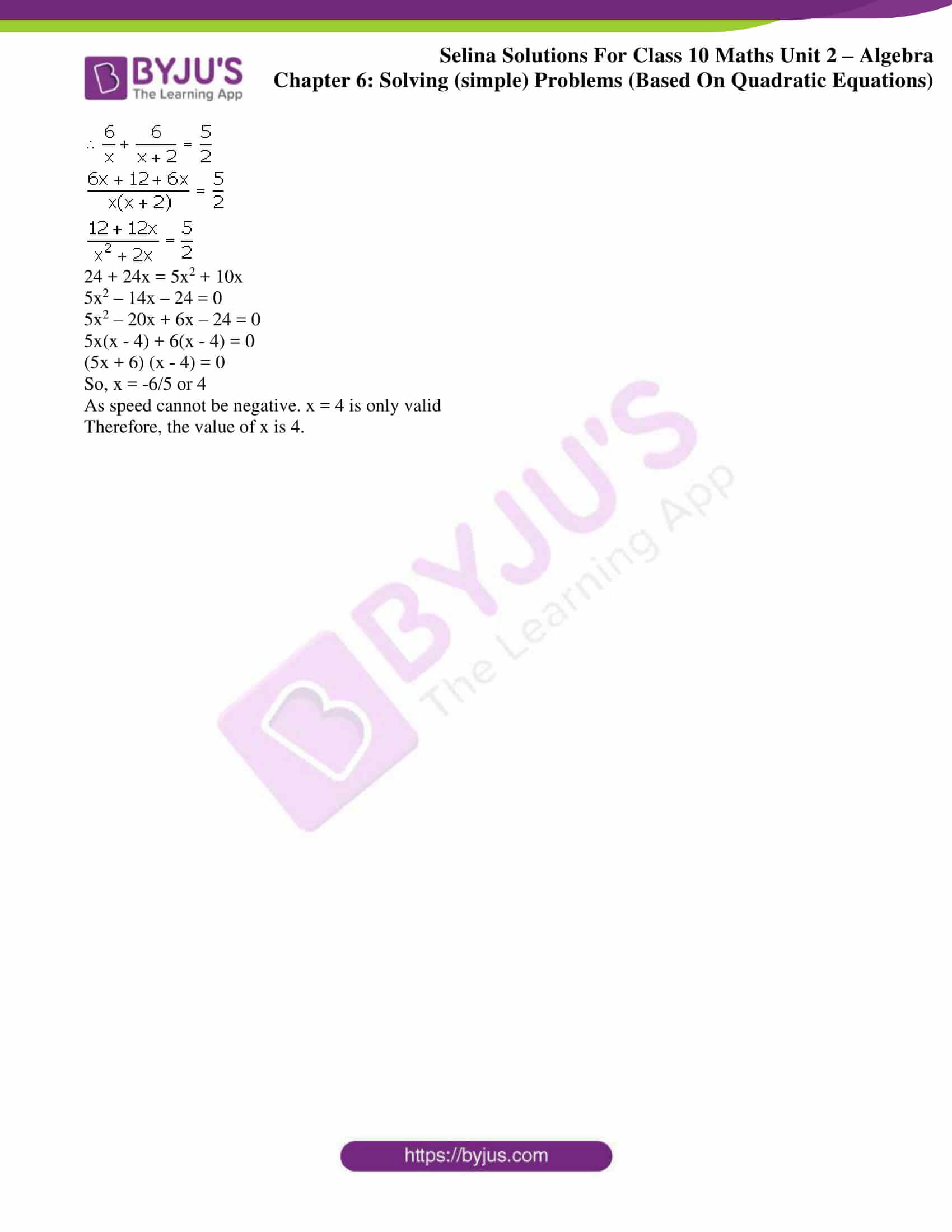# Selina Solutions Concise Maths Class 10 Chapter 6 Solving (simple) Problems (Based On Quadratic Equations) Exercise 6(C)

The distance, speed and time formula is very commonly used in solving several science problems. Based on the same formula, the quadratic equations also find its applications. Students can use the Selina Solutions for Class 10 Maths for any doubt clearance and also strengthen their conceptual knowledge. The solutions to this exercise problems are available in the Concise Selina Solutions for Class 10 Maths Chapter 6 Solving (simple) Problems (Based On Quadratic Equations) Exercise 6(C) PDF in the links here.

## Selina Solutions Concise Maths Class 10 Chapter 6 Solving (simple) Problems (Based On Quadratic Equations) Exercise 6(C) Download PDF### Access Selina Solutions Concise Maths Class 10 Chapter 6 Solving (simple) Problems (Based On Quadratic Equations) Exercise 6(C)

1. The speed of an ordinary train is x km per hr and that of an express train is (x + 25) km per hr.

(i) Find the time taken by each train to cover 300 km.

(ii) If the ordinary train takes 2 hrs more than the express train; calculate speed of the express train.

Solution:

(i) Given,

Speed of the ordinary train = x km/hr

Speed of the express train = (x + 25) km/hr

Distance = 300 km

We know that,

Time = Distance/ Speed

So, the time taken by the ordinary train to cover 300 km = 300/x hrs

And the time taken by the express train to cover 300 km = 300/ (x + 25) hrs

(ii) From the question, it’s given that the ordinary train takes 2 hours more than the express train to cover the distance of 300kms.

Hence, we can write7500 = 2x2 + 50x

2x2 + 50x – 7500 = 0

x2 + 25x – 3750 = 0

x2 + 75x – 50x – 3750 = 0

x(x + 75) – 50(x + 75) = 0

(x – 50) (x + 75) = 0

Thus, x = 50 or -75

As speed cannot be negative we shall ignore x = -75

Therefore,

The speed of the express train = (x + 25) km/hr = 75 km/hr

2. If the speed of a car is increased by 10 km per hr, it takes 18 minutes less to cover a distance of 36 km. Find the speed of the car.

Solution:

Let’s assume the speed of the car to be x km/hr.

Given, distance = 36 km

So, the time taken to cover a distance of 36 km = 36/x hrs  [Since, Time = Distance/ Speed]

And, the new speed of the car = (x + 10) km/hr

So, the new time taken by the car to cover a distance of 36 km = 36/(x + 10) hrs

Then according to the question, we can writex2 + 10x – 1200 = 0

x2 + 40x – 30x – 1200 = 0

x(x + 40) – 30(x + 40) = 0

(x + 40) (x – 30) = 0

Thus,

x = -40 or 30

But, as speed cannot be negative. x = 30 is only considered.

Therefore, the original speed of the car is 30 km/hr.

3. If the speed of an aeroplane is reduced by 40 km/hr, it takes 20 minutes more to cover 1200 km. Find the speed of the aeroplane.

Solution:

Let’s consider the original speed of the aeroplane to be x km/hr.

Now, the time taken to cover a distance of 1200 km = 1200/x hrs  [Since, Time = Distance/ Speed]

Let the new speed of the aeroplane be (x – 40) km/hr.

So, the new time taken to cover a distance of 1200 km = 1200/ (x – 40) hrs

According to the question, we havex(x – 40) = 48000 x 3

x2 – 40x – 144000 = 0

x2 – 400x + 360x – 144000 = 0

x(x – 400) + 360(x – 400) = 0

(x – 400) (x + 360) = 0

As, speed cannot be negative. So we only take, x = 400.

Therefore, the original speed of the aeroplane is 400 km/hr.

4. A car covers a distance of 400 km at a certain speed. Had the speed been 12 km/h more, the time taken for the journey would have been 1 hour 40 minutes less. Find the original speed of the car.

Solution:

Let’s assume x km/h to be the original speed of the car.

We know that,

Time = Distance/ Speed

From the question,

The time taken by the car to complete 400 km = 400/x hrs

Now, when the speed is increased by 12 km.

Increased speed = (x + 12) km/h

And, the new time taken by the car to complete 400 km = 400/(x + 12) hrs

Thus, according to the question we can write4800 x 3 = 5x(x + 12)

5x2 + 60x – 14400 = 0

Dividing by 5 we get,

x2 + 12x – 2880 = 0

x2 + 60x – 48x – 2880 = 0

x(x + 60) – 48(x + 60) = 0

(x + 60) (x – 48) = 0

So, x + 60 or x – 48

x = -60 or 48

As, speed cannot be negative.

x = 48 is only valid

Therefore, the speed of the car is 48 km/h.

5. A girl goes to her friend’s house, which is at a distance of 12 km. She covers half of the distance at a speed of x km/hr and the remaining distance at a speed of (x + 2) km/hr. If she takes 2 hrs 30 minutes to cover the whole distance, find ‘x’.

Solution:

Given,

The girl covers a distance of 6 km at a speed x km/ hr.

So, the time taken to cover first 6 km = 6/x hr [Since, Time = Distance/ Speed]

Also given, the girl covers the remaining 6 km distance at a speed (x + 2) km/ hr.

So, the time taken to cover next 6 km = 6/ (x + 2)

And, the total time taken to cover the whole distance = 2 hrs 30 mins = (120 + 30)/60 = 5/2 hrs

Then the below equation can be formed,24 + 24x = 5x2 + 10x

5x2 – 14x – 24 = 0

5x2 – 20x + 6x – 24 = 0

5x(x – 4) + 6(x – 4) = 0

(5x + 6) (x – 4) = 0

So, x = -6/5 or 4

As speed cannot be negative. x = 4 is only valid

Therefore, the value of x is 4.